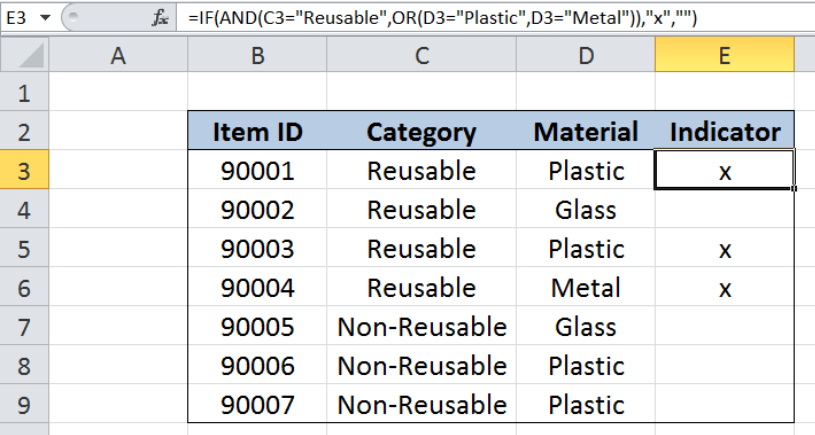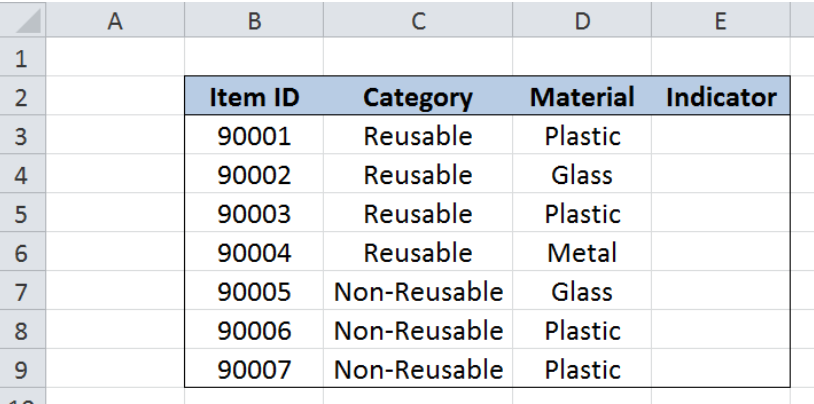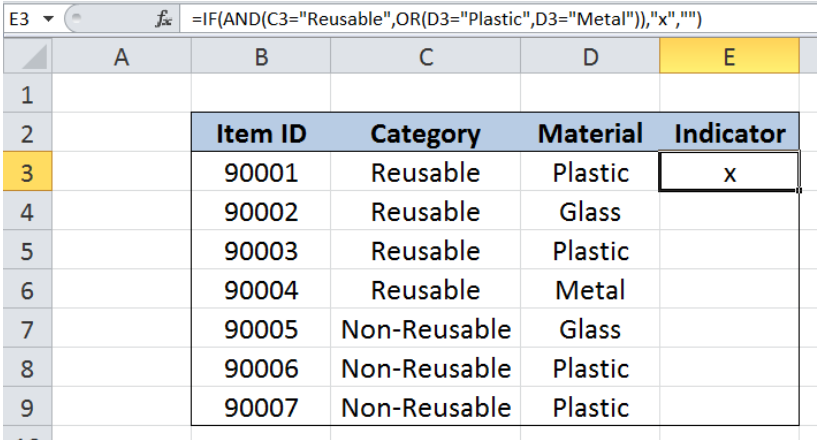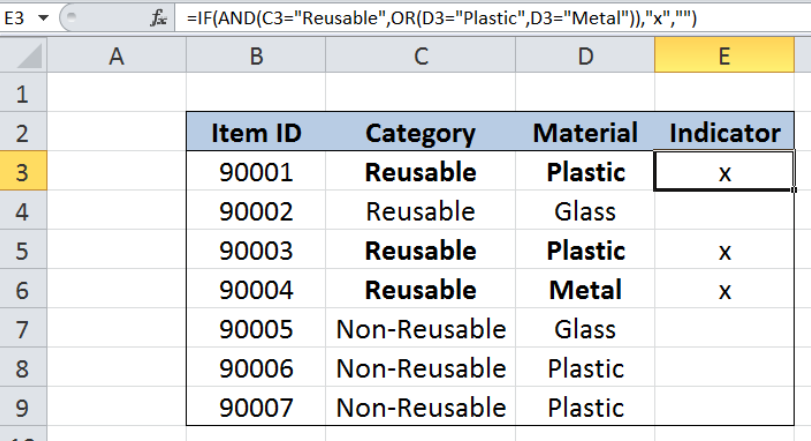Get instant live expert help with Excel or Google Sheets“My Excelchat expert helped me in less than 20 minutes, saving me what would have been 5 hours of work!”

#### Post your problem and you'll get expert help in seconds

Your message must be at least 40 characters
Our professional experts are available now. Your privacy is guaranteed.

# If this AND that OR that

While working with Excel, we are able to evaluate two or more conditions and customize the actions by using the IF, AND and OR functions.  This step by step tutorial will assist all levels of Excel users in marking items in a database that satisfy specific sets of conditions.Figure 1. Final result: IF this AND that OR that

Final formula: `=IF(AND(C3="Reusable",OR(D3="Plastic",D3="Metal")),"x","")`

## Syntax of IF Function

IF function evaluates a given logical test and returns a TRUE or a FALSE

`=IF(logical_test, [value_if_true], [value_if_false])`

• The arguments “value_if_true” and “value_if_false” are optional.  If left blank, the function will return TRUE if the logical test is met, and FALSE if otherwise.

## Syntax of AND Function

AND function evaluates all logical tests and returns a TRUE if all arguments are TRUE; FALSE if one or more arguments is FALSE

`=AND(logical1, [logical2], ...)`

Parameters:

• logical1–  the first condition that we want to test
• only logical1 is required; succeeding conditions are optional

## Syntax of OR Function

OR function evaluates all logical tests and returns TRUE if any argument is TRUE; FALSE if all arguments are FALSE.

`=OR(logical1, [logical2], ...)`

Parameters:

• logical1–  the first condition that we want to test
• only logical1 is required; succeeding conditions are optional

## Setting up Our Data

Our table contains a list of Item ID (column B), Category (column C), Material (column D) and Indicator (column E). In cells E3:E9, we want to indicate whether an item is a “Reusable Plastic” or a “Reusable Metal”.Figure 2. Sample data for IF this AND that

## IF “Reusable” AND “Plastic” OR “Metal”

In order to mark an item if it is both “Reusable” and made of either “Plastic” or “Metal”, we follow these steps:

Step 1.  Select cell E3

Step 2.  Enter the formula: `=``IF(AND(C3="Reusable",OR(D3="Plastic",D3="Metal")),"x","")`

Step 3: Press ENTER

Step 4: Copy the formula in cell E3 to cells E4:E9 by clicking the “+” icon at the bottom right-corner of cell E3 and dragging it down.Figure 3. Entering the formula to mark IF “Reusable” AND made of “Plastic” OR “Metal”

The first part of our formula tests two sets of conditions using the AND function:

• First condition: If the category of an item is “Reusable”
• Second condition: If material is either “Plastic” OR “Metal”

When both conditions are satisfied, the IF function returns the character “x”, which marks the item as a “Reusable Plastic” or “Reusable Metal”.  Otherwise, it returns an empty string “”.

As a result, our formula returns an “x” in cells E3, E5 and E6, which satisfy our two conditions.Figure 4. Output: Mark “x” IF “Reusable” AND made of “Plastic” OR “Metal”

Most of the time, the problem you will need to solve will be more complex than a simple application of a formula or function. If you want to save hours of research and frustration, try our live Excelchat service! Our Excel Experts are available 24/7 to answer any Excel question you may have. We guarantee a connection within 30 seconds and a customized solution within 20 minutes.

### Did this post not answer your question? Get a solution from connecting with the expert.Another blog reader asked this question today on Excelchat:## Subscribe to Excelchat.coAnother blog reader asked this question today on Excelchat: